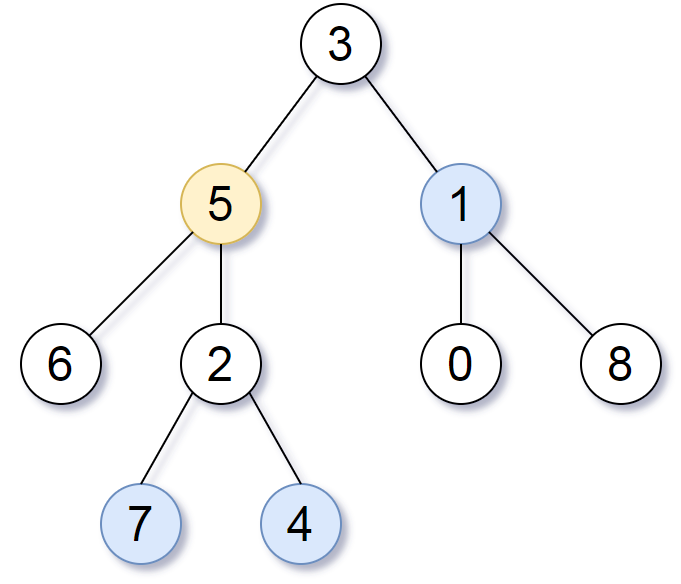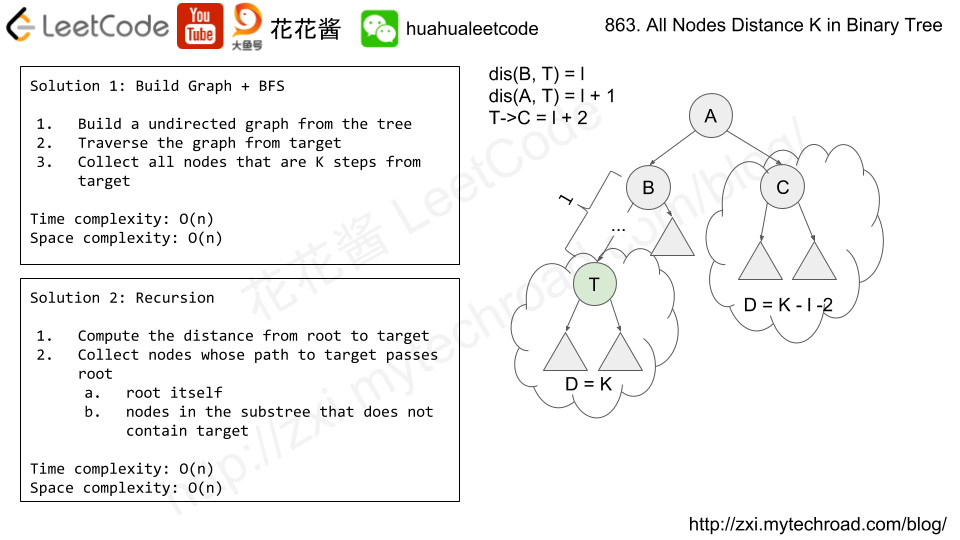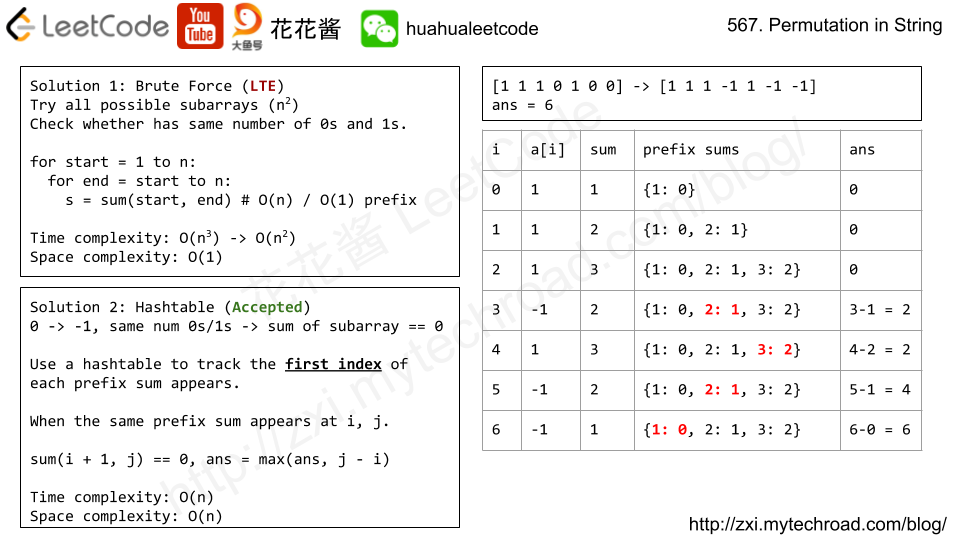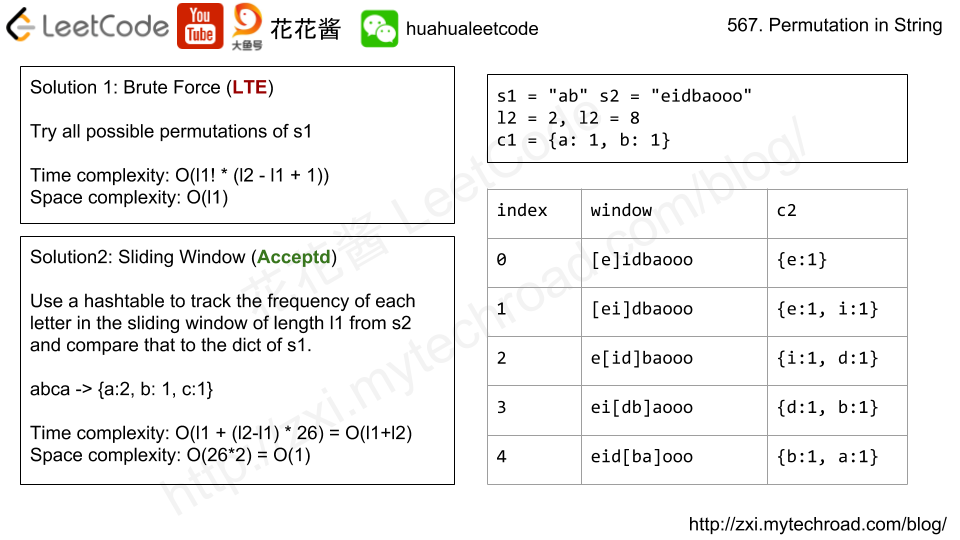Press "Enter" to skip to content

# Problem

We are given a binary tree (with root node root), a target node, and an integer value K.

Return a list of the values of all nodes that have a distance K from the target node.  The answer can be returned in any order.

Example 1:

Input: root = [3,5,1,6,2,0,8,null,null,7,4], target = 5, K = 2
Output: [7,4,1]
Explanation:
The nodes that are a distance 2 from the target node (with value 5)
have values 7, 4, and 1.Note that the inputs "root" and "target" are actually TreeNodes.
The descriptions of the inputs above are just serializations of these objects.



Note:

1. The given tree is non-empty.
2. Each node in the tree has unique values 0 <= node.val <= 500.
3. The target node is a node in the tree.
4. 0 <= K <= 1000.# Solution1: DFS + BFS

Use DFS to build the graph, and use BFS to find all the nodes that are exact K steps from target.

Time complexity: O(n)

Space complexity: O(n)

C++

Array version

# Solution 2: Recursion

Recursively compute the distance from root to target, and collect nodes accordingly.

Time complexity: O(n)

Space complexity: O(n)

# Problem

At a lemonade stand, each lemonade costs $5. Customers are standing in a queue to buy from you, and order one at a time (in the order specified by bills). Each customer will only buy one lemonade and pay with either a $5$10, or $20 bill.  You must provide the correct change to each customer, so that the net transaction is that the customer pays $5. Note that you don’t have any change in hand at first. Return true if and only if you can provide every customer with correct change. Example 1: Input: [5,5,5,10,20] Output: true Explanation: From the first 3 customers, we collect three$5 bills in order.
From the fourth customer, we collect a $10 bill and give back a$5.
From the fifth customer, we give a $10 bill and a$5 bill.
Since all customers got correct change, we output true.


Example 2:

Input: [5,5,10]
Output: true


Example 3:

Input: [10,10]
Output: false


Example 4:

Input: [5,5,10,10,20]
Output: false
Explanation:
From the first two customers in order, we collect two $5 bills. For the next two customers in order, we collect a$10 bill and give back a $5 bill. For the last customer, we can't give change of$15 back because we only have two \$10 bills.
Since not every customer received correct change, the answer is false.


# Solution: Simulation + Greedy

Always use 10 bill first.

Time complexity: O(n)

Space complexity: O(1)

C++

# Problem

Given a binary array, find the maximum length of a contiguous subarray with equal number of 0 and 1.

Example 1:

Input: [0,1]
Output: 2
Explanation: [0, 1] is the longest contiguous subarray with equal number of 0 and 1.


Example 2:

Input: [0,1,0]
Output: 2
Explanation: [0, 1] (or [1, 0]) is a longest contiguous subarray with equal number of 0 and 1.


Note: The length of the given binary array will not exceed 50,000.# Solution: HashTable

Prefix sum + hashtable

Time complexity: O(n)

Space complexity: O(n)

V2: Using array instead of a hashtable

Time complexity: O(2*n + 1 + n)

Space complexity: O(2*n + 1)

# Problem

https://leetcode.com/problems/permutation-in-string/description/

Given two strings s1 and s2, write a function to return true if s2 contains the permutation of s1. In other words, one of the first string’s permutations is the substring of the second string.

Example 1:

Input:s1 = "ab" s2 = "eidbaooo"
Output:True
Explanation: s2 contains one permutation of s1 ("ba").


Example 2:

Input:s1= "ab" s2 = "eidboaoo"
Output: False


Note:

1. The input strings only contain lower case letters.
2. The length of both given strings is in range [1, 10,000].# Solution: Sliding Window

Time Complexity: O(l1 + l2 * 26) = O(l1 + l2)

Space Complexity: O(26 * 2) = O(1)

C++

# Problem

Suppose you have a long flowerbed in which some of the plots are planted and some are not. However, flowers cannot be planted in adjacent plots – they would compete for water and both would die.

Given a flowerbed (represented as an array containing 0 and 1, where 0 means empty and 1 means not empty), and a number n, return if n new flowers can be planted in it without violating the no-adjacent-flowers rule.

Example 1:

Input: flowerbed = [1,0,0,0,1], n = 1
Output: True


Example 2:

Input: flowerbed = [1,0,0,0,1], n = 2
Output: False


Note:

1. The input array won’t violate no-adjacent-flowers rule.
2. The input array size is in the range of [1, 20000].
3. n is a non-negative integer which won’t exceed the input array size.

# Solution: Greedy

Time complexity: O(n)

Space complexity: O(1)

C++

Mission News Theme by Compete Themes.